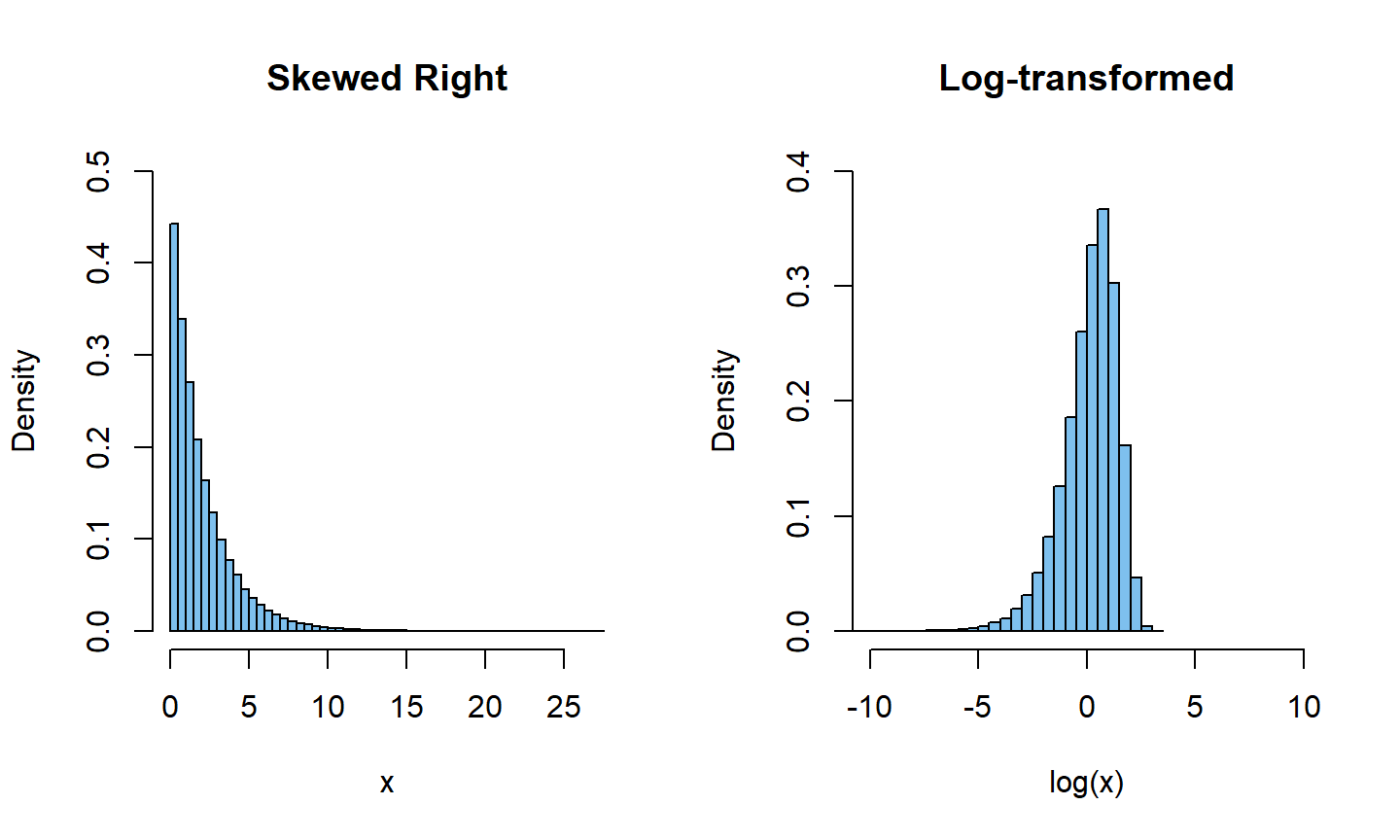Spring 2018

## Stem and Leaf Plots

In a stem-and-leaf plot, each number is broken into two parts -

• The first part is called the stem and consists of the beginning digit(s).

• The second part is called the leaf and consists of the final digit(s).

The stems are written in a column in ascending order, and the leaves that match up with those stems are written on the corresponding row.

A stem-and-leaf plot of the number of characters in 50 emails.

 22 0 64 10 6 26 25 11 4 14 7 1 10 2 7 5 7 4 14 3 1 5 43 0 0 3 25 1 9 1 2 9 0 5 3 6 26 11 25 9 42 17 29 12 27 10 0 0 1 1

## Stem and Leaf Plots

First, sort the data in ascending order.

   0  0  0  0  0  0  1  1  1  1  1  2  2  3  3  3  4  4  5  5  5  6  6
  7  7  7  9  9  9 10 10 10 11 11 12 14 14 16 17 22 25 25 25 26 26 27
 29 42 43 64
  The decimal point is 1 digit(s) to the right of the |

0 | 00000011111223334455566777999
1 | 0001124467
2 | 25556679
3 |
4 | 23
5 |
6 | 4

## Split Stem and Leaf Plots

When there are too many numbers on one row or there are only a few stems, we split each row into two halves, with the leaves from 0-4 on the first half and the leaves from 5-9 on the second half. The resulting graph is called a split stem-and-leaf plot.

  The decimal point is 1 digit(s) to the right of the |

0 | 000000111112233344
0 | 55566777999
1 | 00011244
1 | 67
2 | 2
2 | 5556679
3 |
3 |
4 | 23
4 |
5 |
5 |
6 | 4

## Dot Plot

A dot plot uses dots to show the frequency, or number of occurrences, of the values in a data set. The higher the stack of dots, the greater the number of occurrences there are of the corresponding value.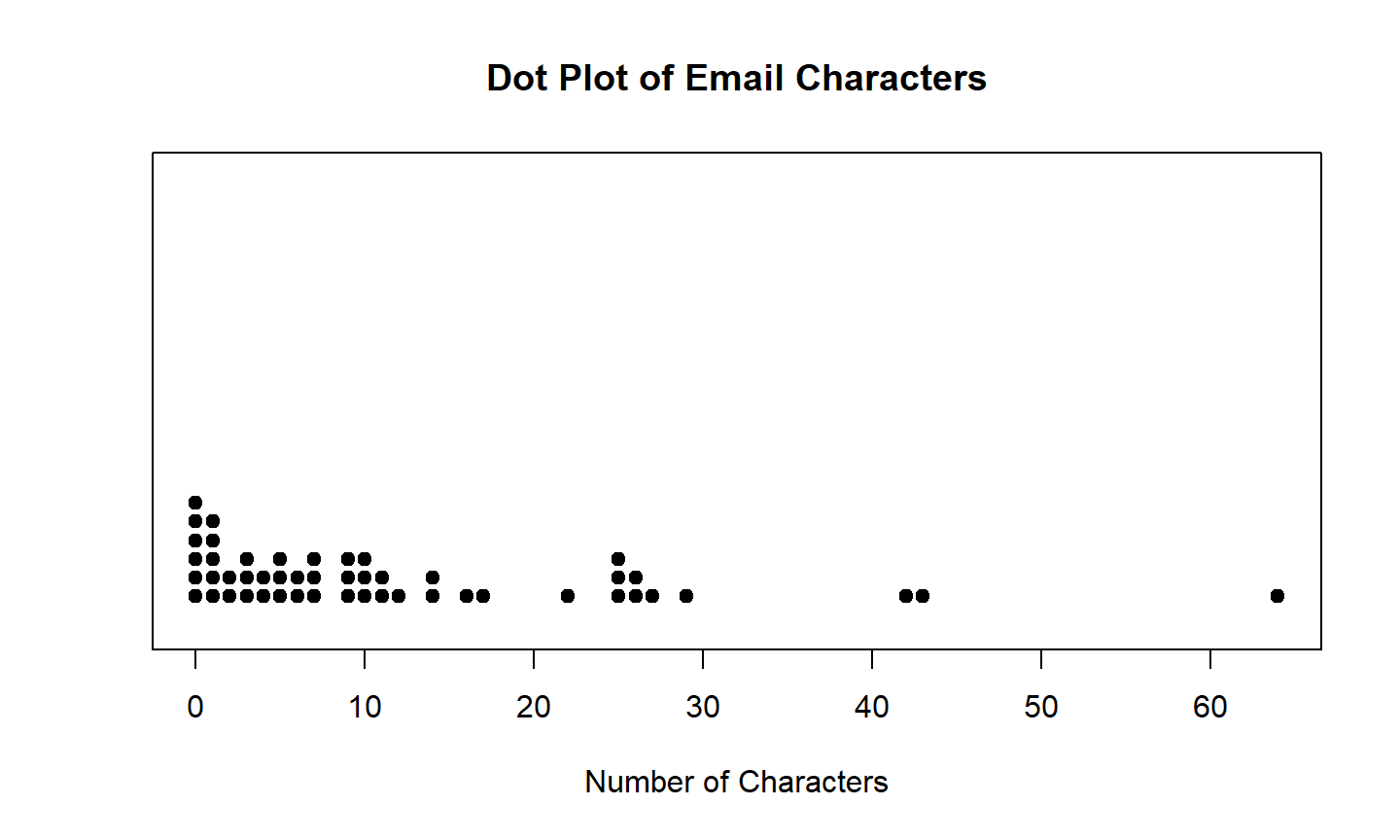## Histogram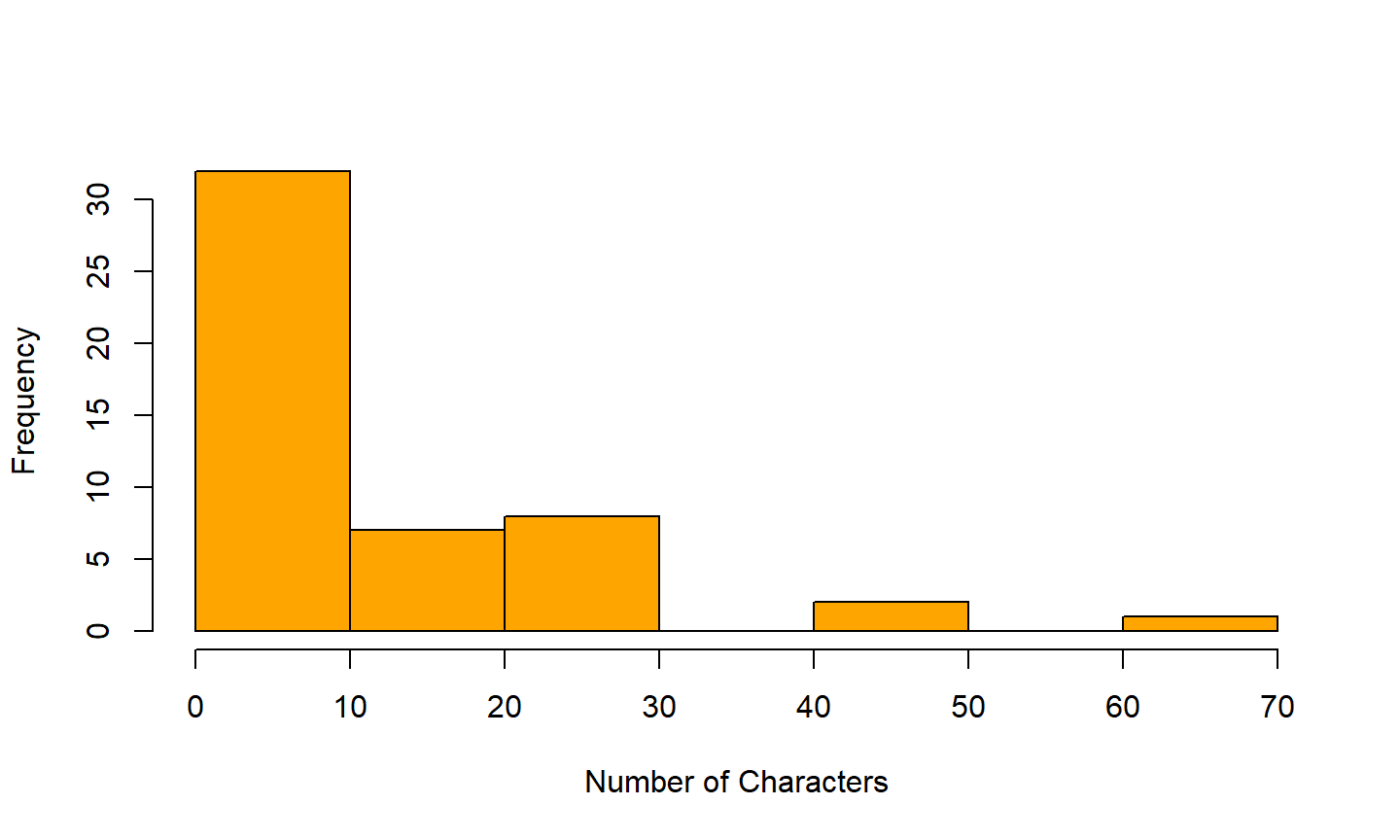## Cumulative Frequency Histogram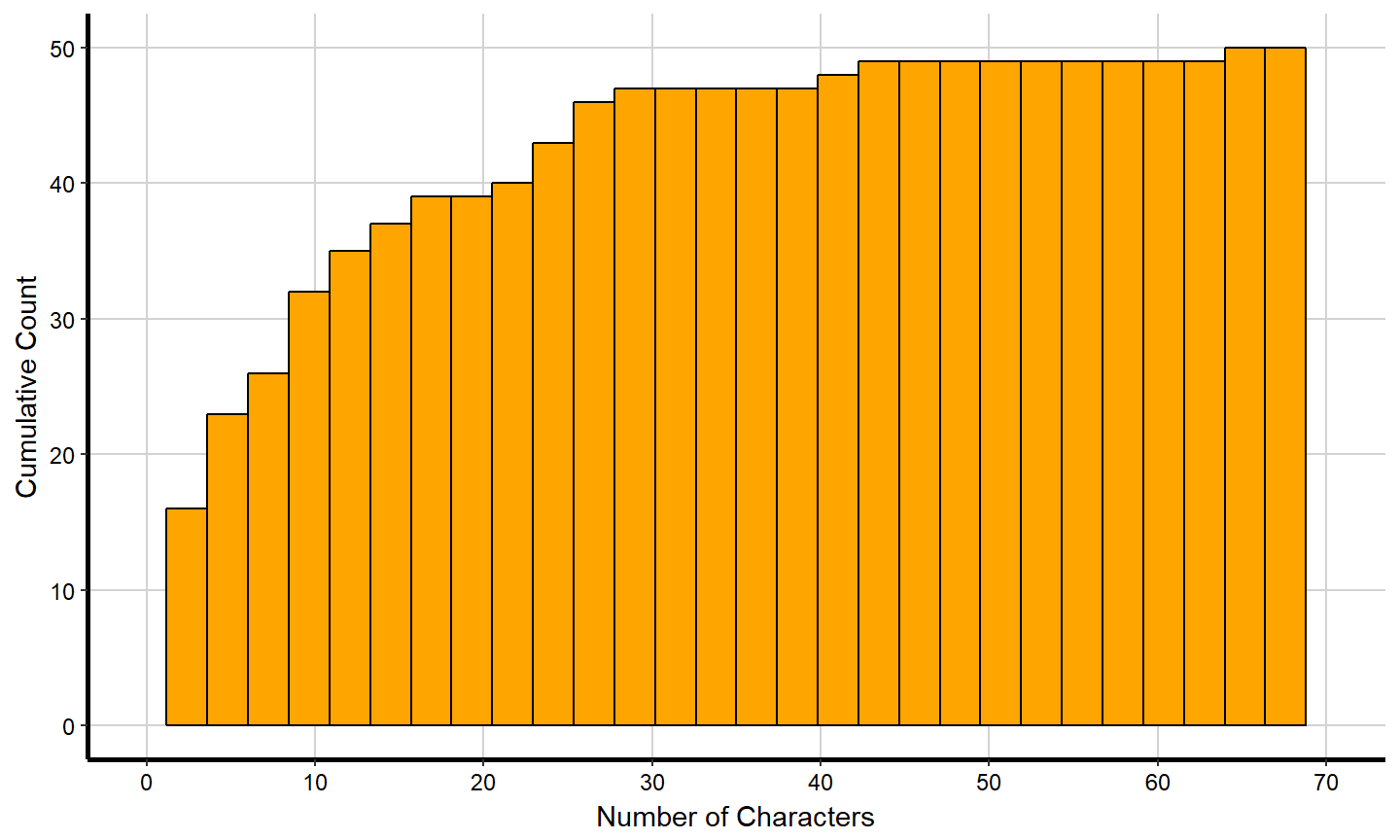## Modes

Mode is represented by a prominent peak in the distribution.

1. Unimodel Distribution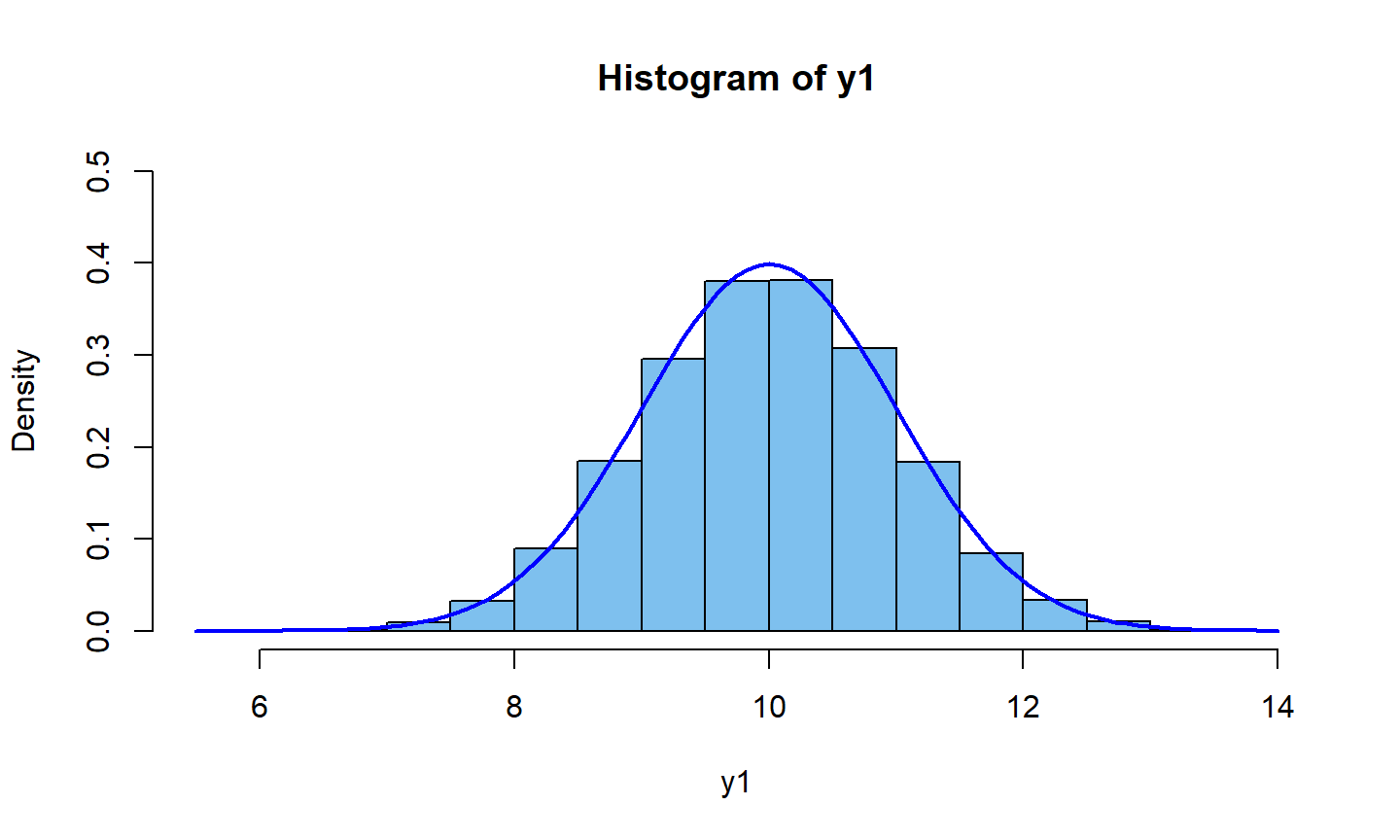## Modes

1. Bimodal Distribution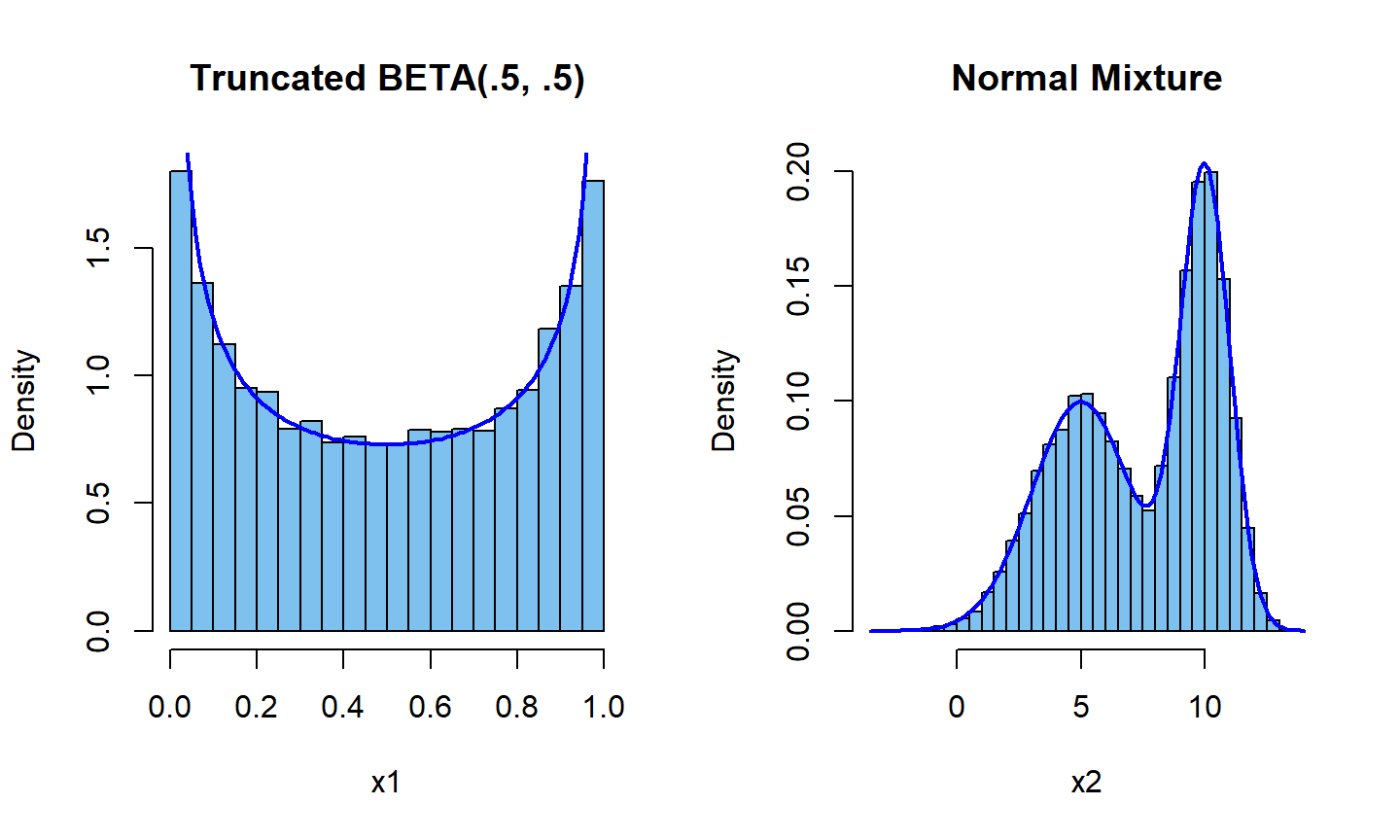## Modes

1. Multimodal Distribution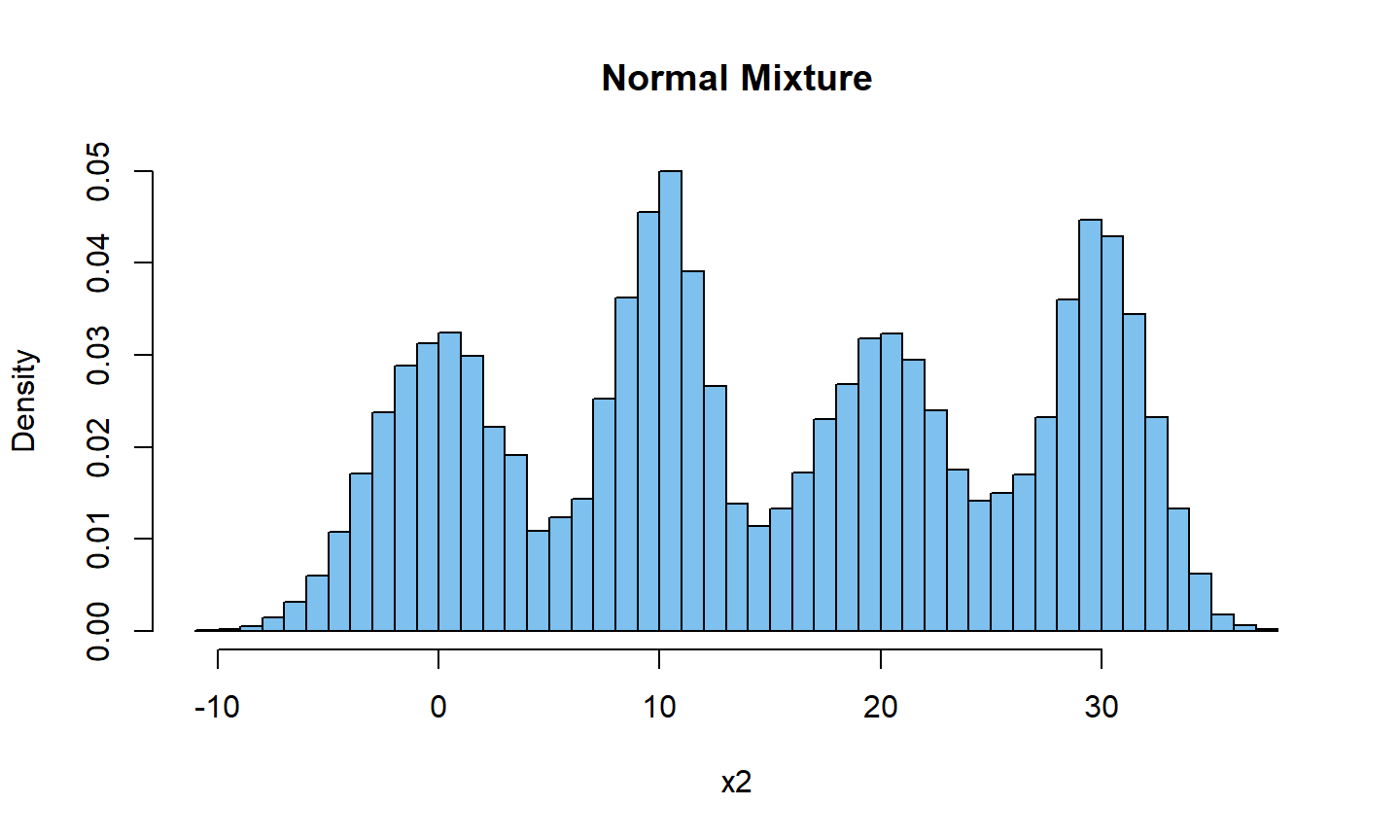## Modes

1. Uniform Distribution

* All the bins have the same frequency, or at least close to the same frequency.
* It is a distribution without a mode.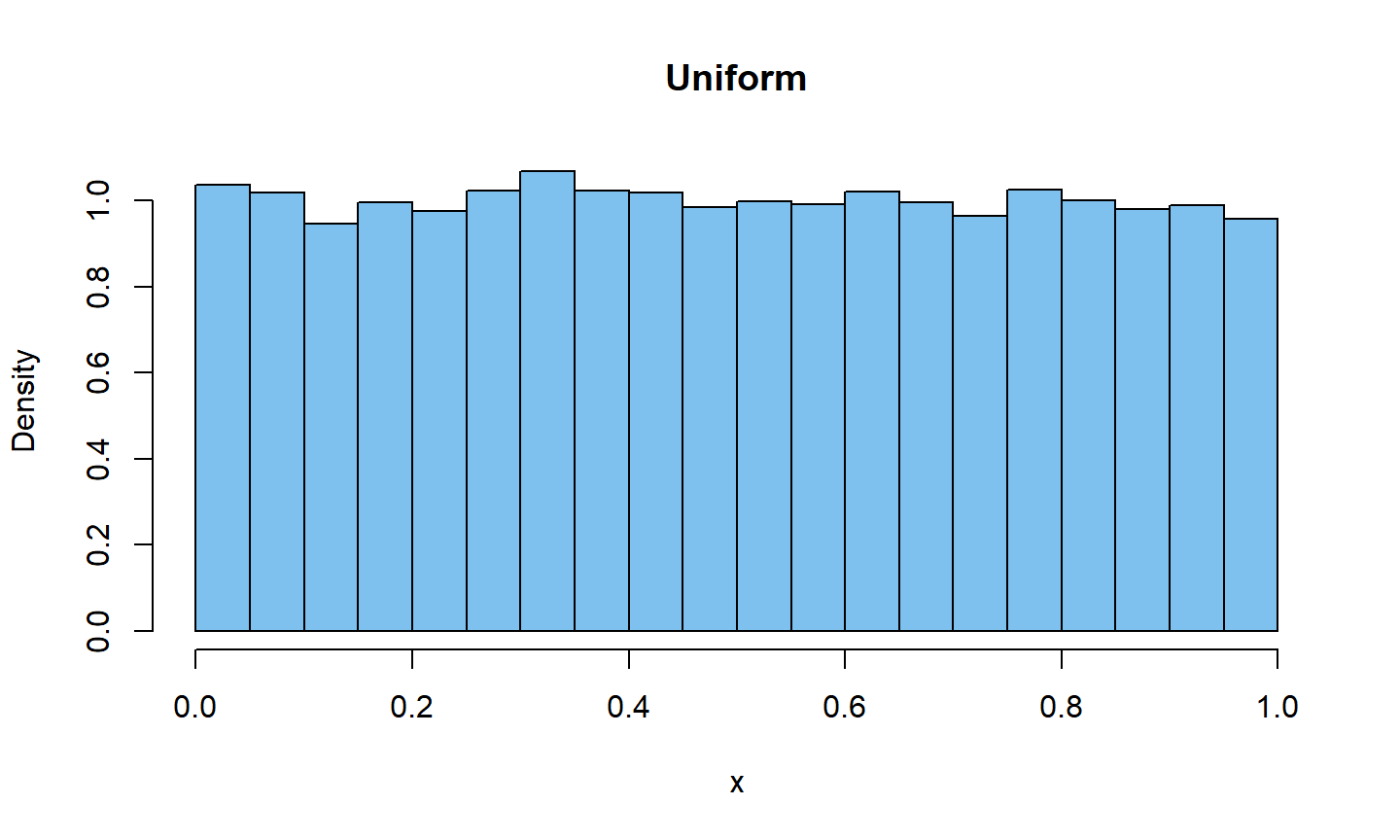## Symmetry

The histogram for a symmetric distribution will look the same on the left and the right of its center.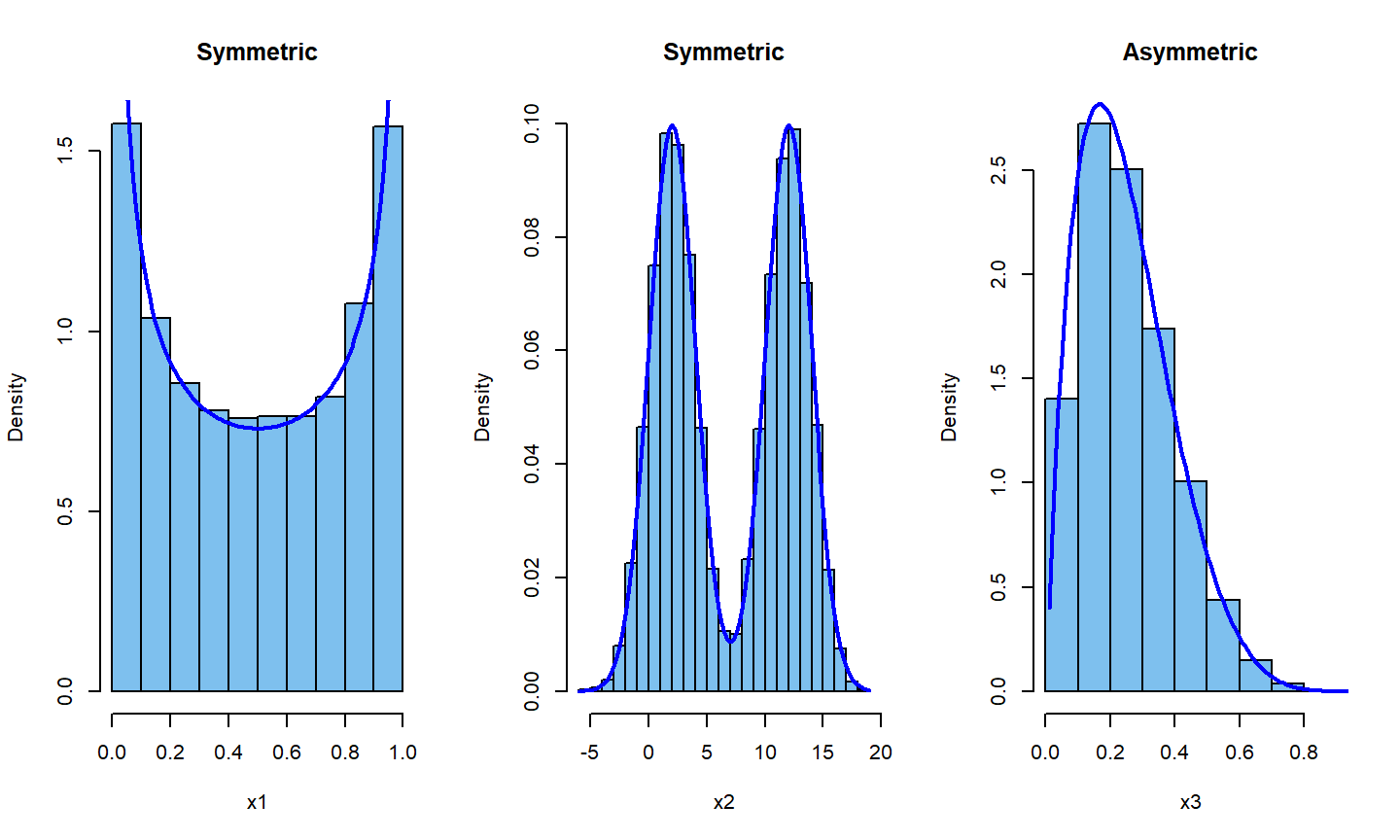## Skew

• A histogram is skewed right if the longer tail is on the right side of the mode.
• A histogram is skewed left if the longer tail is on the left side of the mode.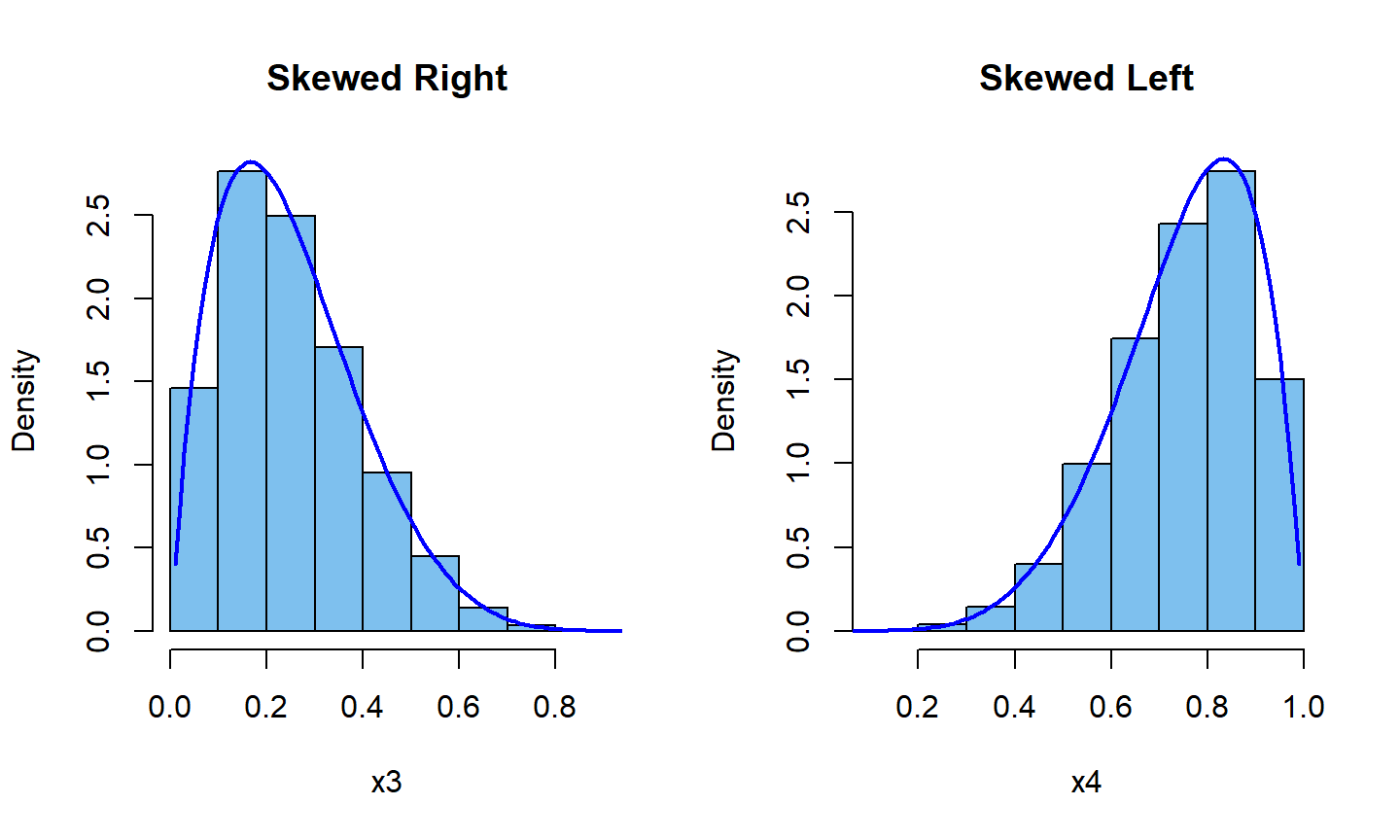## Outlier

• An Outlier is a data value that is far above or far below the rest of the data values.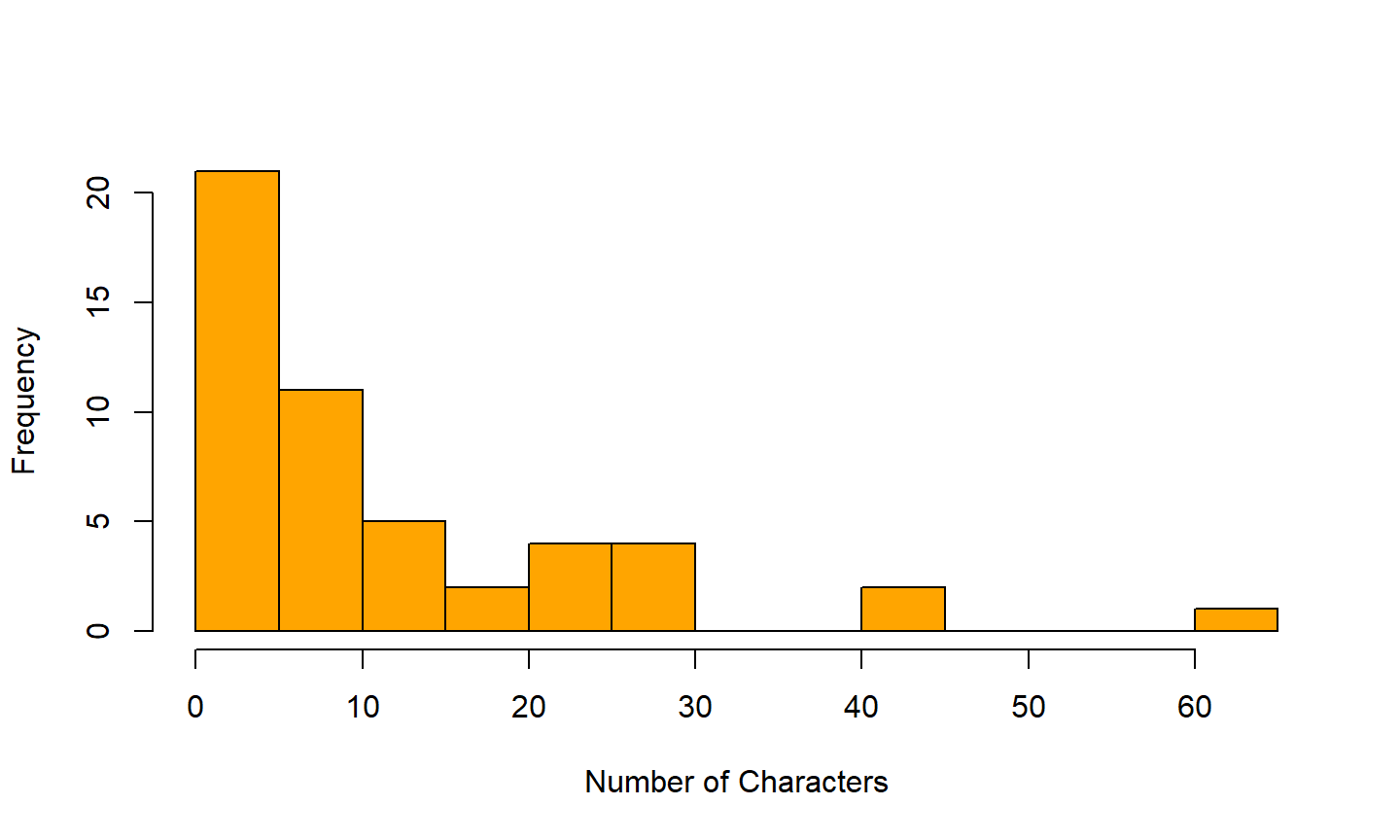## Mean and Median

Mean

The sample mean of a numerical variable is computed as the sum of all of the observations divided by the number of observations:

##### $$\bar x = \sum_{i=1}^n x_i = \frac{(x_1+x_2+...+x_n)}{n}$$

The mean follows the tail

• In a right skewed distribution, the mean is greater than the median.
• In a left skewed distribution, the mean is less than the median.
• In a symmetric distribution, the mean and median are approximately equal.

Median: the number in the middle

The median splits an ordered data set in half. If there are an even number of observations, the median is the average of the two middle values. If there are an odd number of observations, the median is the middle value.

## Mean and Median

   0  0  0  0  0  0  1  1  1  1  1  2  2  3  3  3  4  4  5  5  5  6  6
  7  7  7  9  9  9 10 10 10 11 11 12 14 14 16 17 22 25 25 25 26 26 27
 29 42 43 64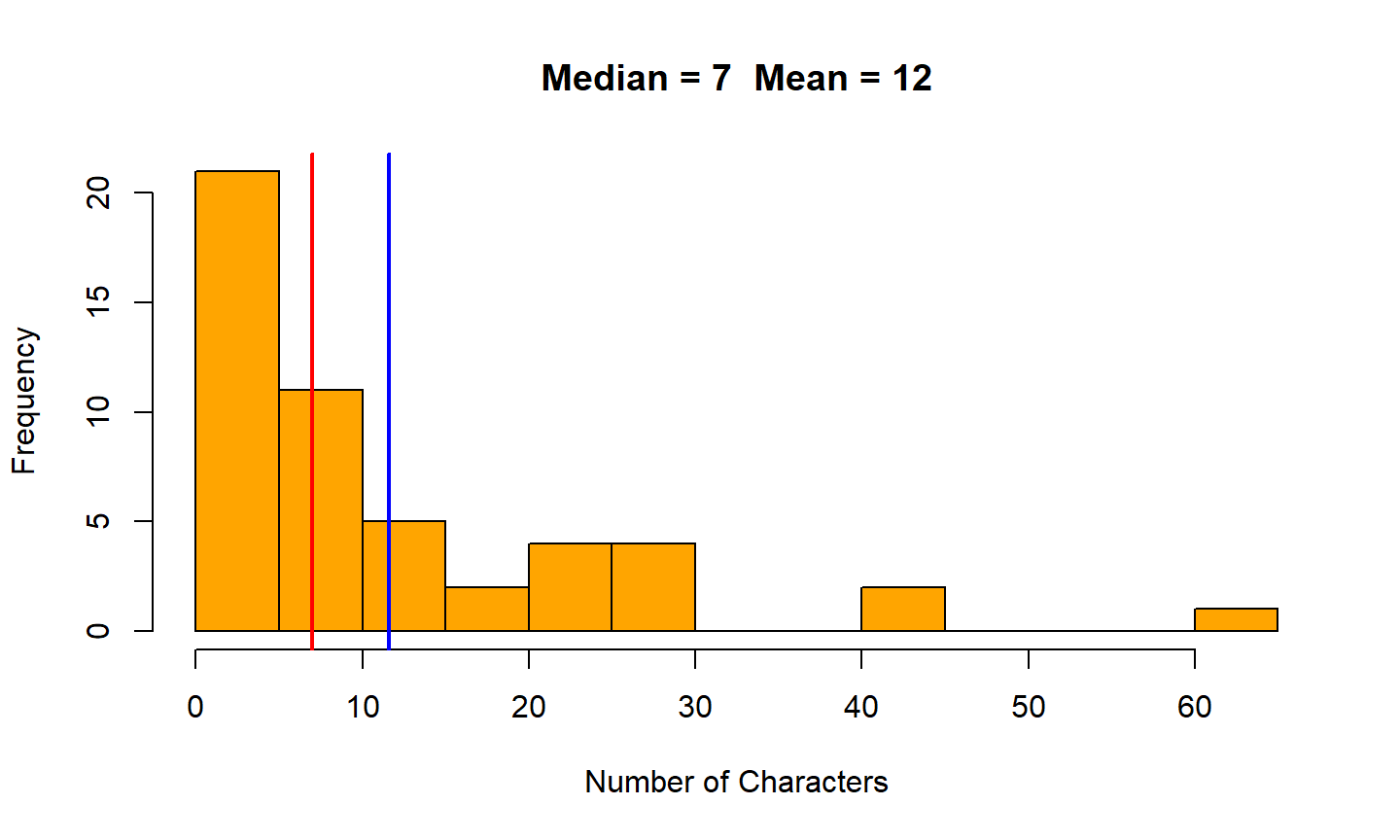## Calculating the Median

#### $$n$$ is odd

1. Sort the series in ascending order.
2. If the series has odd number $$(n)$$ of entries, the median is at position $$\frac{n+1}{2}.$$
3. Find the median of the series: $$2,4,5,(6),7,9,9$$
4. The median is $$6.$$

#### $$n$$ is even

1. Sort the series in ascending order.
2. If the series has even number $$(n)$$ of entries, the median is the average of the two middle numbers: $$\frac{n}{2},\frac{n+1}{2}.$$
3. Find the median of the numbers: $$2,2,4,6,7,8$$
4. Median is the average of the third and the fourth numbers: $$\frac{4+6}{2}=5$$

## Mean Vs. Median

##### List: $${2,3,3,4}$$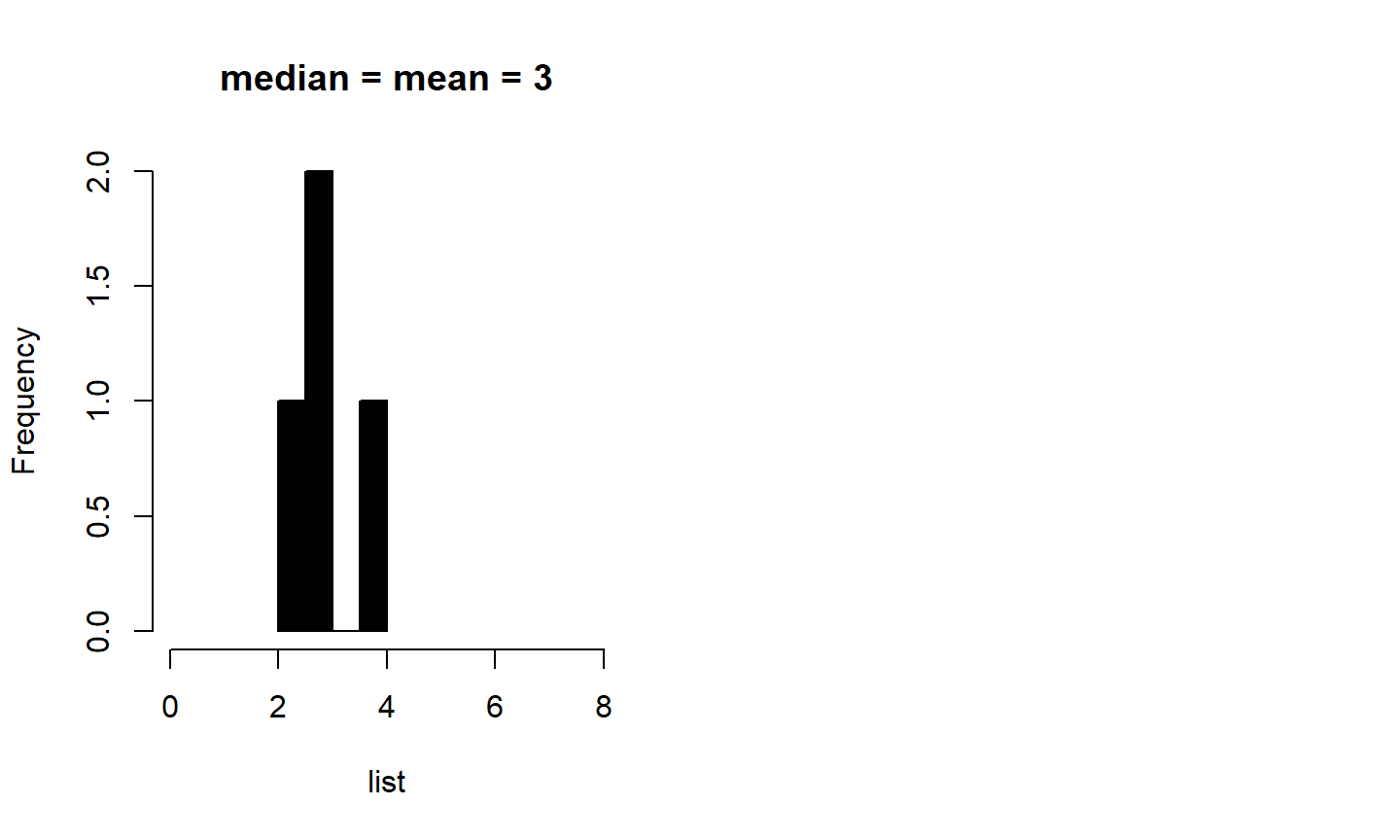##### List: $$2,3,3,7$$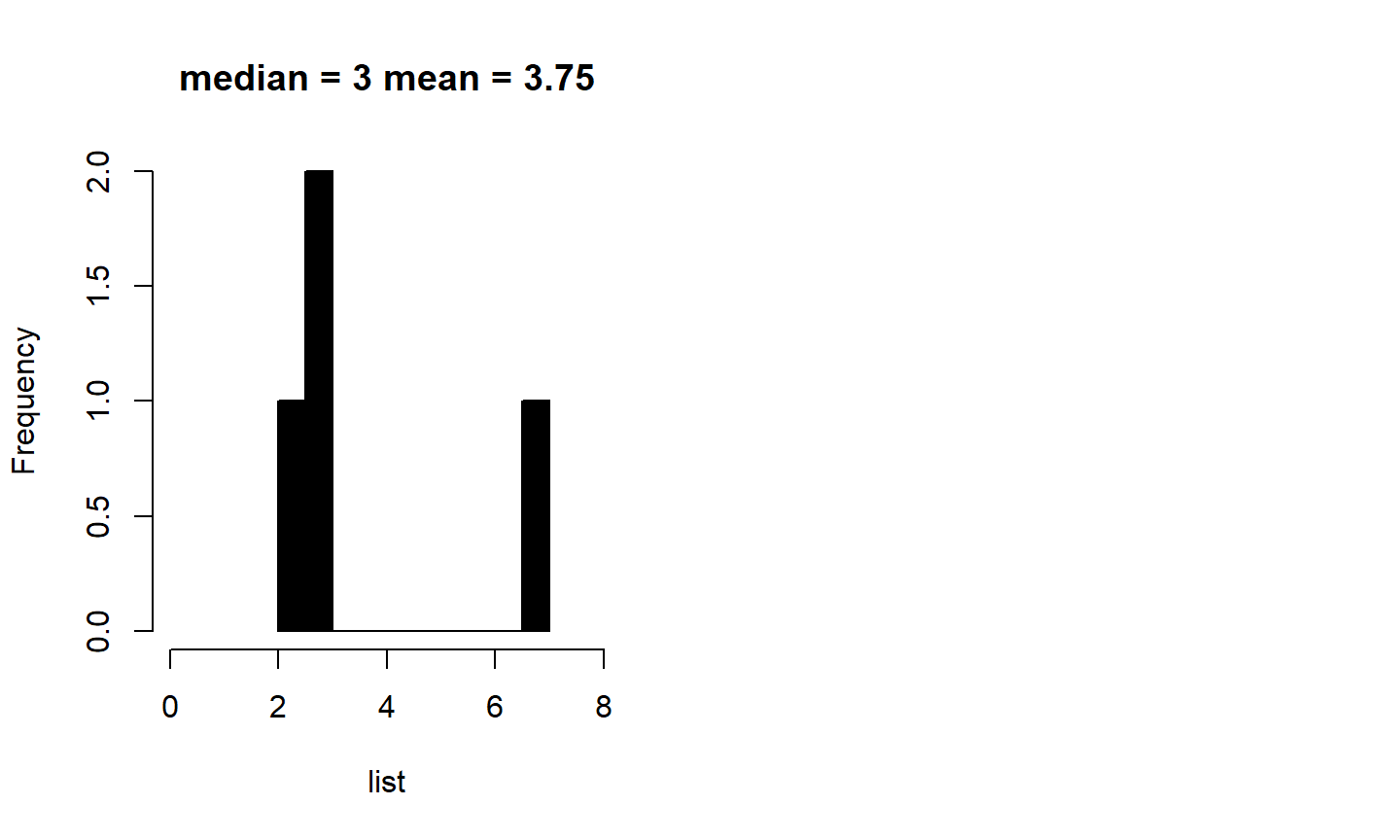The median is unaffected by outlier.

## Weighted Mean

The weighted mean is the same as the mean, except that it is influenced more by some observations than others. We assign weights to observations as a sort of way of describing its relative importance.

The weighted mean of observations $$x_1, x_2,...,x_n$$ using weights $$w_1, w_2,...,w_n$$ is given by

##### $$\bar x =\frac{w_1x_1+w_2x_2+...+w_nx_n}{w_1+w_2+...+w_n}$$

The simple mean is a weighted mean where all the weights are 1.

## Range

The range is the difference between the maximum and minimum values.

$$range = maximum - minimum$$

The range is sensitive to outliers. A single high or low value will affect the range significantly.

## Percentiles and Quartiles

• Percentiles divide the data in one hundred groups.
• The $$n^{th}$$ percentile is the data value such that $$n^{th}$$ percent of the data lies below that value.

Three Quartiles $$(Q_1, Q_2, Q_3)$$

• $$Q_1$$ represents the first quartile, which is the 25th percentile, and is the median of the smaller half of the data set.
• $$Q_2$$ represents the second quartile, which is equivalent to the 50th percentile (i.e. the median).
• $$Q_3$$ represents the third quartile, or 75th percentile, and is the median of the larger half of the data set
• Interquartile Range $$(IQR) = Q_3 - Q_1$$

Outliers in the context of a box plot

When in the context of a box plot, define an outlier as an observation that is more than $$1.5 \times IQR$$ above $$Q_3$$ or $$1.5 \times IQR$$ below $$Q_1$$. Such points are marked using a dot or asterisk in a box plot.

## Box Plot

Data: $$[5, 5, 9, 10, 15, 16, 20, 30, 40]$$

   Min. 1st Qu.  Median    Mean 3rd Qu.    Max.
5.00    9.00   15.00   16.67   20.00   40.00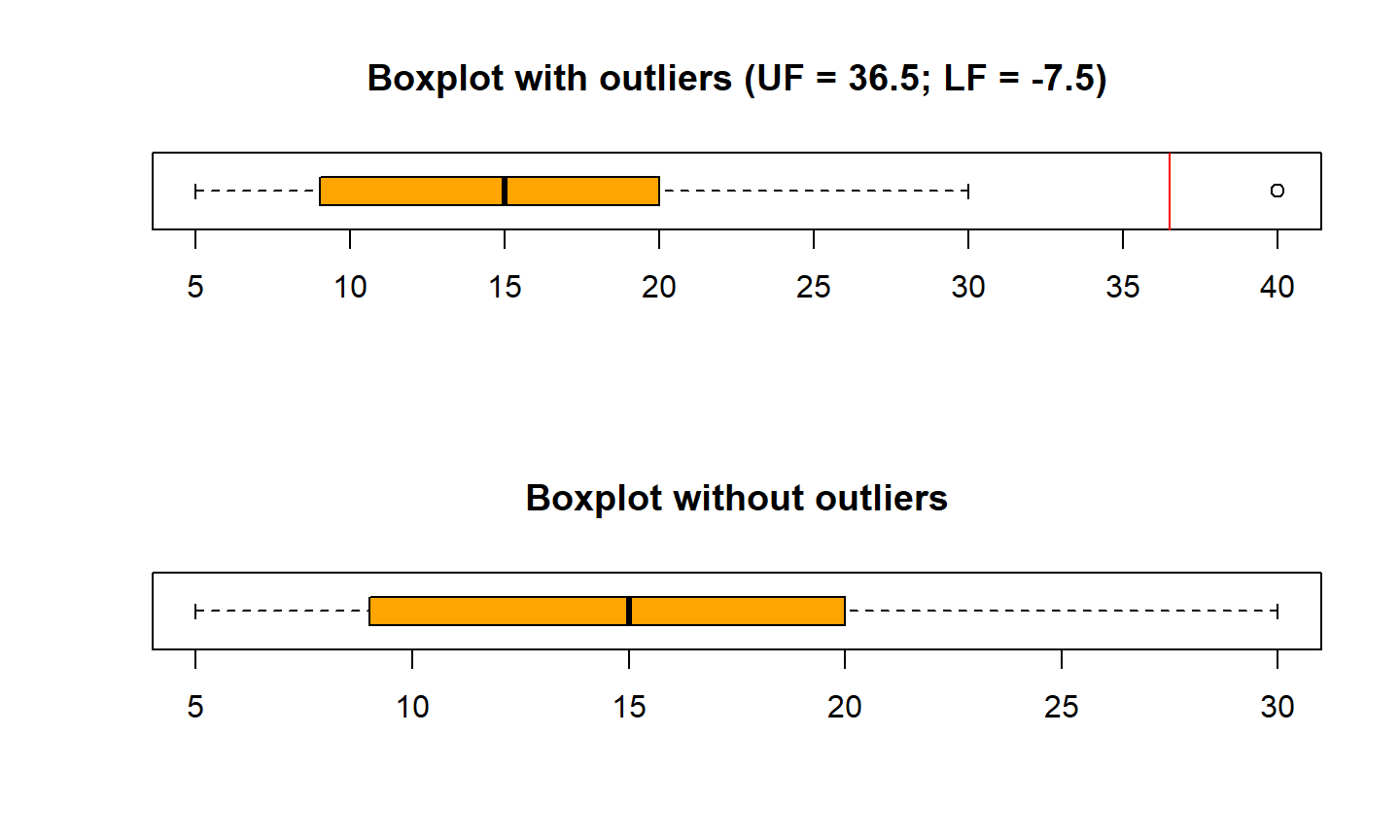## Standard Deviation

• SD describes how far away, on average, the observations are from the mean.
• In other word, SD describes the variability of the data set within the range of the dataset.
• Low variability or small spread means that the values tend to be more clustered together.
• High variability or large spread means that the values tend to be far apart.

Calculating the Standard Deviation

The standard deviation is the square root of the variance. It is roughly the average distance of the observations from the mean.

$\bbox[yellow,5px] { \color{black}{s= \sqrt{\frac{1}{n-1}\sum(x_i-\bar x)^2}} }$

$$Calculate \space SD \space of \space [0,1]$$

## Which histogram has the largest SD?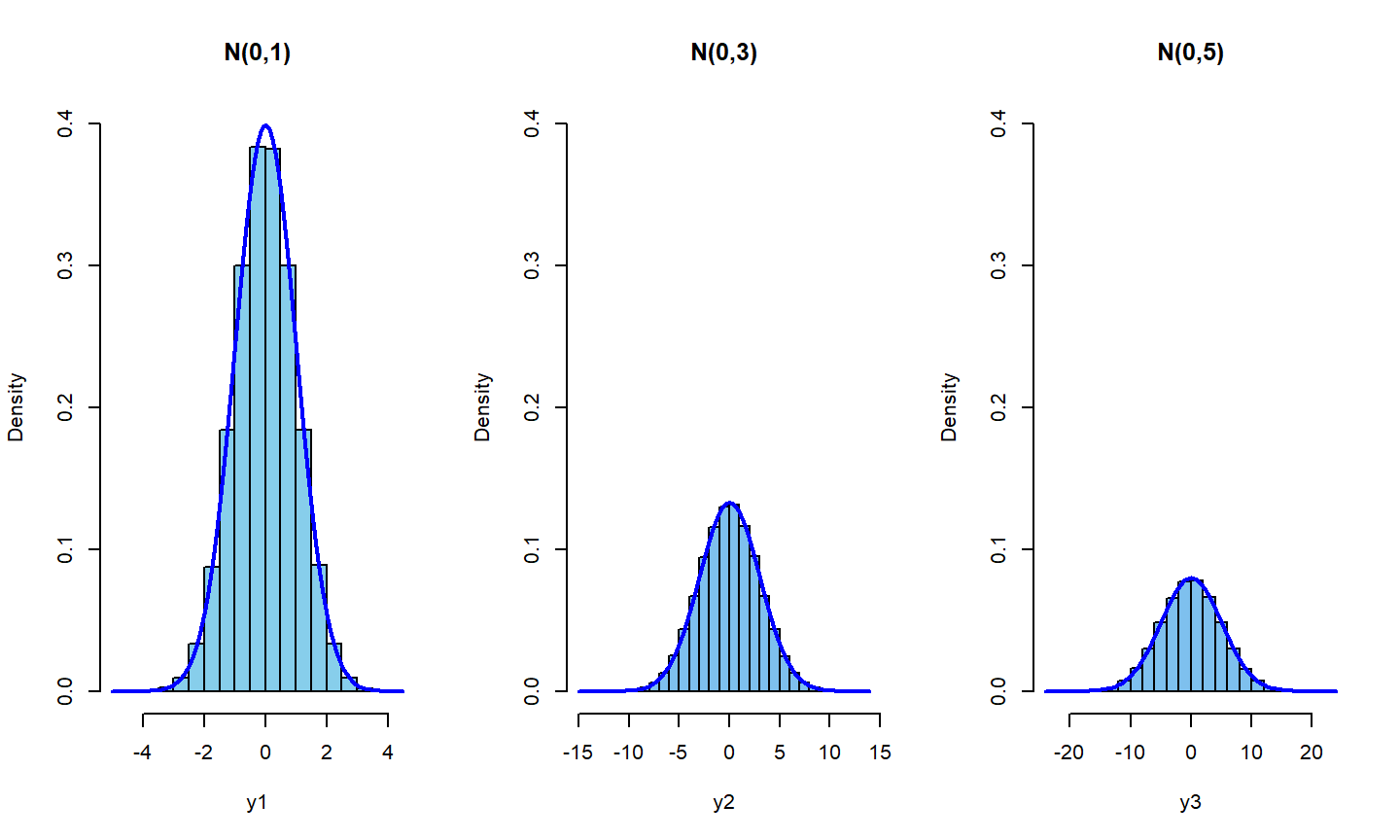Notice the spread of the distributions.

## Altering SD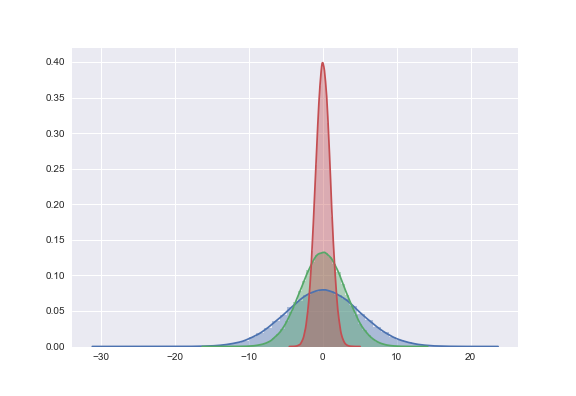## Group Comparison

Comparing distributions of median household income for counties by population gain status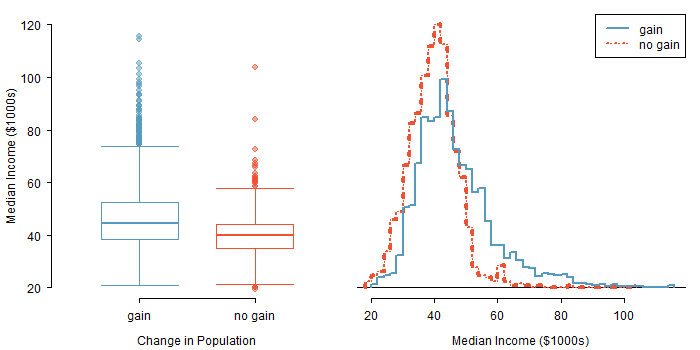Source: OpenIntroOrg

## Smoothing Plot: Moving Average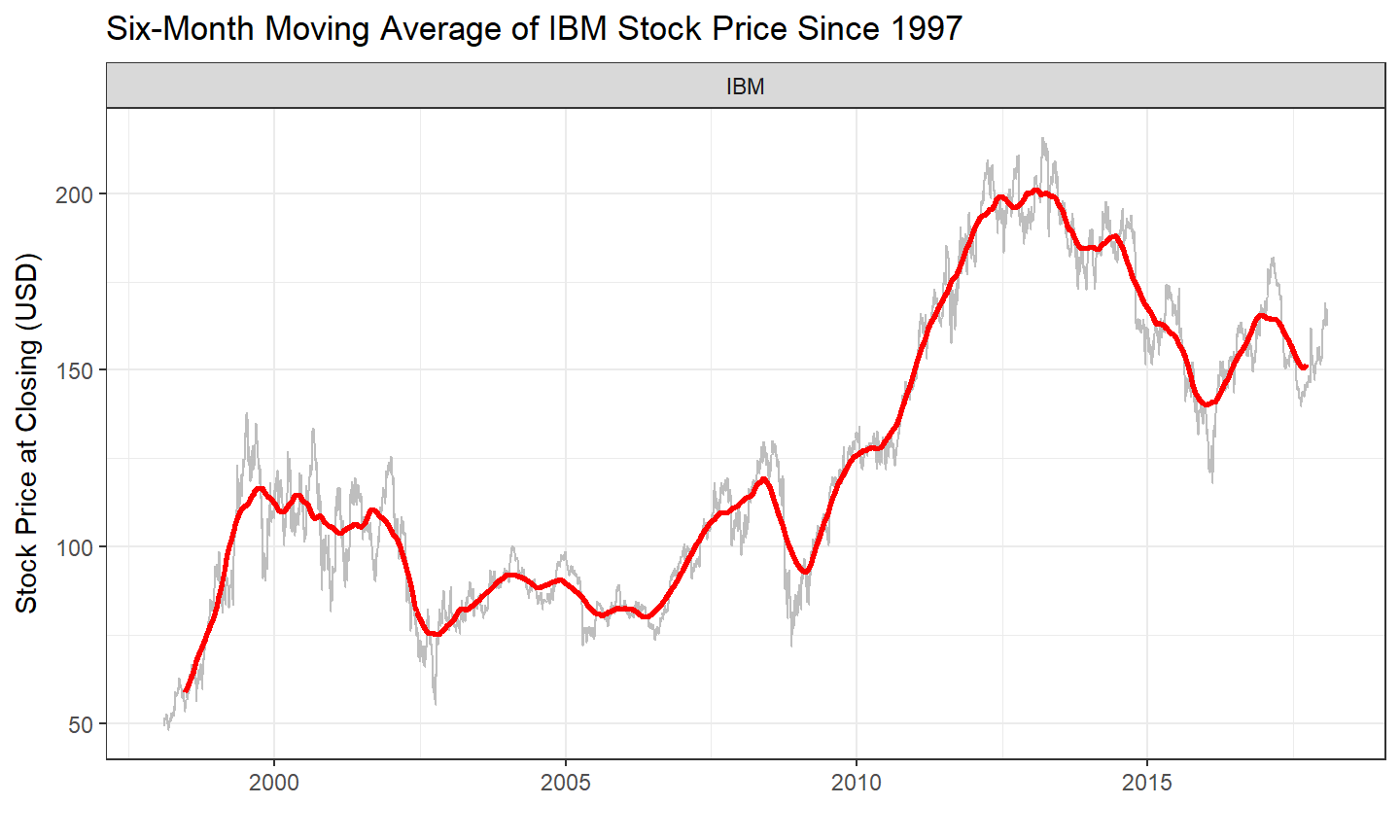## Transformation of the Data

• Data with outliers (i.e. skewed distribution) are hard to interpret
• Such data are often transformed into their logarithmic form to give the skewed distribution a normal (i.e. roughly unimodal and symmetric) shape for better interpretation